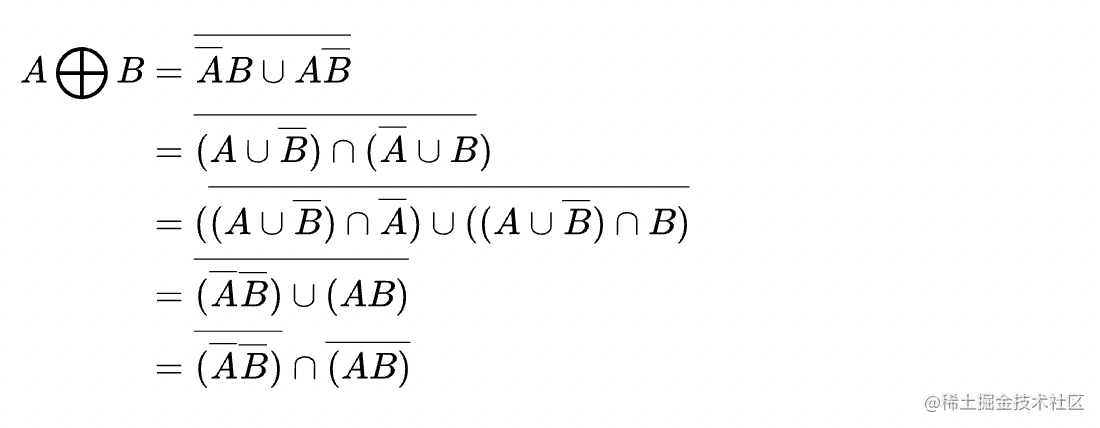# 371. 两整数之和

### 思路分析

``````0 + 0 = 0
0 + 1 = 1
1 + 0 = 1
1 + 1 = 0 (进位)

``````0 + 0 = 0
0 + 1 = 0
1 + 0 = 0
1 + 1 = 1

``````int getSum(int a, int b) {
while (b != 0) {
int carry = (int)(a & b) << 1;
a = a ^ b;
b = carry;
}
return a;
}

### 总结

1， 在不使用异或的情况下表示a^b``````1.(a|b)&(~a|~b)
2.~(~a&~b)&~(a&b)
3.(a&~b)|(~a&b)

``````int bitXor(int x, int y) {
return ~(~x&~y)&~(x&y);
}